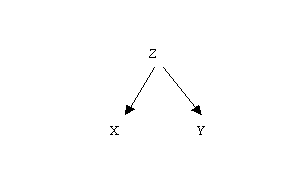Lecture 16
Correlation and Causation

I'd like to make a few comments about correlation and causation. It is often said that a correlation between two variables does not imply causation. This is generally true. Just because the amount of time spent working on an exam is positively correlated with the grade on the exam does not mean that spending more time on an exam will necessarily lead to a better grade. The two might be correlated for some other reason.

In some instances, the adage about correlation and causation is actually not true. Consider the situation in which we have a well conducted experiment that compares two groups. We compute a correlation coefficient and there is a significant relationship between the experimental variable (i.e., group membership) and the dependent variable. This would, of course, be the same as conducting a t-test. Assuming we have eliminated any confounds and we used random assignment, we should be able to conclude that the independent variable caused the dependent variable. So in this case a correlation between the independent variable and the dependent variable indicates that the independent variable caused the dependent variable.

If a non-experimental study is conducted, there is likely to be some alternative explanations for a relationship between the independent and the dependent variable, because we did not randomly assign participants to experimental conditions.

A Couple of Common Alternative Explanations for a Correlation Between X and Y
There are three general reasons we cannot conclude that X caused Y just because X and Y are correlated (in cases where X is not experimentally manipulated). First, we don't know for sure that Y did not cause X. I'll use arrows to indicate causation.

X ----> Y (X causes Y)

but, it might be that Y causes X as in the following diagram:

Y -----> X (Y causes X)

Second, we don't know that they don't cause each other:Finally, there is often a third variable that might cause both X and Y as this diagram points out:The latter is referred to as the "third variable problem". My favorite example of the third variable problem is the correlation between the number of fire hydrants in a city and the number of dogs in a city. Cities with more fire hydrants tend to have more dogs. Why the relationship? A third variable. Any guesses what the third variable might be?